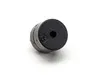# Avoid Injuries with Smart Backpack

Smart Backpack helps you to avoid injuries caused by backpacks by alerting you about improper uses.

IntermediateFull instructions provided3 hours5,541

## Things used in this project

### Hardware componentsArduino 101
×1
 SparkFun Force Sensitive Resistor
×3Buzzer
×1

### Software apps and online servicesBlynkArduino IDE

## Code

### Arduino101.ino

Arduino
```#define BLYNK_PRINT Serial

#include <EEPROM.h>
#include "CurieIMU.h"
#include <BlynkSimpleCurieBLE.h>
#include <CurieBLE.h>
#include <Wire.h>
#include <SPI.h>

WidgetTerminal terminal(V2);

#define center A0                  //Define Sensor pins
#define left A1
#define right A2
#define buz 2

char auth[] = "########";          //Enter your Blynk Authorization Code Here
BLEPeripheral  blePeripheral;

int cal_weight;
int body_weight;
int fc,fr,fl;
int mr,ml,mc,cr,cl,cc;
int tempr,templ,tempc;
int cal_rec=0;
int mem;
int val;
int x,y,z,thx,thy,thz;
int pin1;
int mode = 0;

for(int i=0;i<50;i++){
delay(2);
}
fc=fc/50;
fr=fr/50;
fl=fl/50;
thx = thx/50;   //Get the average values of the readings
thy = thy/50;
thz = thz/50;
}

void calibrate(){
while(mode == 1){}

if (mode == 2){

tempr = fr;
templ = fl;     //Save the values of FSRs
tempc = fc;
}

while (mode ==3){}

if (mode == 5){

mr = (fr - tempr)/100;
ml = (fl-templ)/100;
mc = (fc-tempc)/100;       //Calculate threshold values
cr = 1000-(mr*tempr);
cl = 1000-(ml*templ);
cc = 1000-(mc*tempc);
x = thx;
y = thy;
z = thz;

EEPROM.write(0,mr);
EEPROM.write(1,ml);
EEPROM.write(2,mc);     //Write threshold values to EEProm
EEPROM.write(3,cr);
EEPROM.write(4,cl);
EEPROM.write(5,cc);
EEPROM.write(6,x);
EEPROM.write(7,y);
EEPROM.write(8,z);

}

}

void active(){

fc = fc*mc + cc;//Calculate forces on FSRs using pre-saved threshold values
fl = fl*ml + cl;
fr = fr*mr + cr;

if(abs(fl-fr)>300) {
tone(buz,300);                //Alerts if straps are unbalanced
terminal.println("Straps are unbalanced");
}
if(fc> 500) {
tone(buz,600);                       //Alerts if the backpack is overfilled
terminal.println("Harmful force on the spine.");
}
if(fl+fr>cal_weight){
tone(buz,1400);             //Alerts if the backpack is over-weight
terminal.println("Too heavy");
}

tone(buz,2000);
terminal.println("Harmful posture detected");
}
delay(500);
digitalWrite(buz,LOW);
}

void setup(){
pinMode(3,OUTPUT);
pinMode(left,INPUT);
pinMode(right,INPUT);          //Setting the sensor pin modes
pinMode(center,INPUT);
Serial.begin(9600);

blePeripheral.setLocalName("Arduino101Blynk");
blePeripheral.setDeviceName("Arduino101Blynk");
blePeripheral.setAppearance(384);

Blynk.begin(auth, blePeripheral);

blePeripheral.begin();
}

void loop(){
Blynk.run();
blePeripheral.poll();
}

BLYNK_WRITE(V0){                    //Read the changes in the weight slider
body_weight = param.asInt();
cal_weight = body_weight/10;  // Calculate the safe carrying weight
}

BLYNK_WRITE(V1){                               // Going to calibrate mode
pin1 = param.asInt();
if (pin1 == 1){
mode = mode+1;
calibrate();
}

}
BLYNK_WRITE(V2){
if(mode==2){
terminal.print("Put 1kg weight and Press Enter");
mode = mode+1;
}                   // Terminal Notifications in the calibration process
if(mode==3){
terminal.println("Values Saved");
terminal.println("Put 2kg weight and Press Enter");
mode = mode+1;
}
if(mode==6){
terminal.println("Values Saved");
terminal.println("Calibration Succesfull");
mode = 0;
active();
}
}

BLYNK_WRITE(V3){                                          // Enter button
int pin3 = param.asInt();
if (pin3 == 1){
mode = mode+1;
}
}

BLYNK_WRITE(V4){                                // Turn On/Off the buzzer
int pin4 = param.asInt();
if (pin4 == 1){
tone(buz , 1000);
}
if (pin4 == 0){
digitalWrite(buz , LOW);
}
}
BLYNK_WRITE(V5){                                  //Turning on the device
int pinValue = param.asInt();

if (pinValue == 1){
while(pinValue == 1){
int pinValue = param.asInt();

active();

}
}
}
```

## Credits

### Chathuranga Liyanage

10 projects • 52 followers
Entrepreneur | Founder - SRQ Robotics | Roboticist